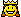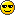# Table after Eastbourne Borough##Table after Eastbourne Borough

Attendance 783

Congratulations to the two people who got 50 points this week . . . . . Ben E and Claire K   (How do you do it ?)
Only narrowly missing out on the 100 points for an exact matchWe lesser mortals (further down the table) can only bask in your reflected gloryBenE     55 + 50  = 105
Claire K  30 + 50 = 80
Ma Gibbons   55 + 10 = 65
Bristol Mike    55 + 0 = 55
Comrade Powell   15 + 20 = 35
Midsomer-Chris   25 + 10 = 35
Rabbit  20 +15 = 35
Ashley 30 + 0 = 30
LB    25 + 0 = 25
Sean     15 + 10 =25
Kermit   15 + 0 = 15
Luton Roman    15 + 0 = 15
Beau Nash   10 + 0 = 10
Midsomer-Steve    10 + 0 = 10
Azlimey 0 + 0 = 0
Maurice Ashman 0
PhilB1973   0 + 0 = 0
2Weirdtown   0 + 0 = 0Midsomer-chris

Posts : 917
Join date : 2014-02-21
Age : 61Permissions in this forum:
You cannot reply to topics in this forum